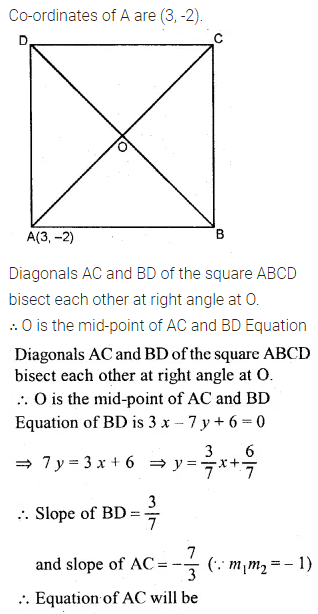# ML Aggarwal Class 10 Solutions for ICSE Maths Chapter 12 Equation of a Straight Line Chapter Test

## ML Aggarwal Class 10 Solutions for ICSE Maths Chapter 12 Equation of a Straight Line Chapter Test

ML Aggarwal Class 10 Solutions for ICSE Maths Chapter 12 Equation of a Straight Line Chapter Test

Question 1.
Find the equation of a line whose inclination is 60° and the y-intercept is -4.
Solution:Question 2.
Write down the gradient and the intercept on the y-axis of the line 3y + 2x = 12.
Solution:Question 3.
If the equation of a line is y – √3x + 1, find its inclination.
Solution:Question 4.
If the line y = mx + c passes through the points (2, -4) and (-3, 1), determine the values of m and c.
Solution:Question 5.
If the point (1, 4), (3, -2) and (p, – 5) lie on a straight line, find the value of p.
Solution: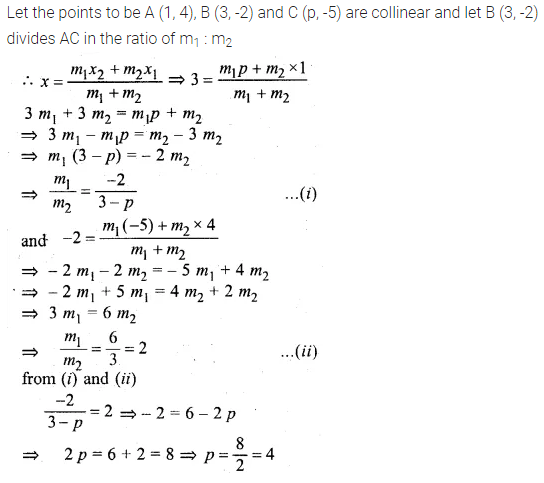Question 6.
Find the inclination of the line joining the points P (4, 0) and Q (7, 3).
Solution:Question 7.
Find the equation of the line passing through the point of intersection of the lines 2x + y = 5 and x – 2y = 5 and having y-intercept equal to $$– \frac { 3 }{ 7 }$$
Solution: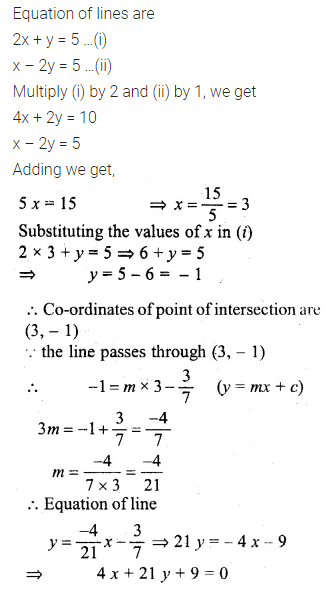Question 8.
If point A is reflected in the y-axis, the co-ordinates of its image A1, are (4, -3),
(i) Find the co-ordinates of A
(ii) Find the co-ordinates of A2, A3 the images of the points A, A1, Respectively under reflection in the line x = -2
Solution:Question 9.
If the lines $$\frac { x }{ 3 } +\frac { y }{ 4 } =7$$ and 3x + ky = 11 are perpendicular to each other, find the value of k.
Solution:Question 10.
Write down the equation of a line parallel to x – 2y + 8 = 0 and passing through the point (1, 2).
Solution: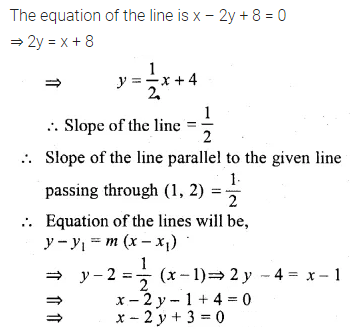Question 11.
Write down the equation of the line passing through (-3, 2) and perpendicular to the line 3y = 5 – x.
Solution:Question 12.
Find the equation of the line perpendicular to the line joining the points A (1, 2) and B (6, 7) and passing through the point which divides the line segment AB in the ratio 3 : 2.
Solution:Question 13.
The points A (7, 3) and C (0, -4) are two opposite vertices of a rhombus ABCD. Find the equation of the diagonal BD.
Solution: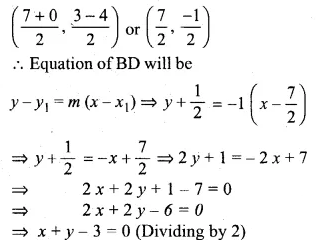Question 14.
A straight line passes through P (2, 1) and cuts the axes in points A, B. If BP : PA = 3 : 1, find: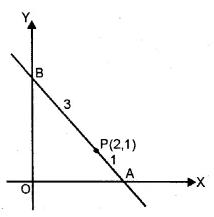(i) the co-ordinates of A and B
(ii) the equation of the line AB
Solution: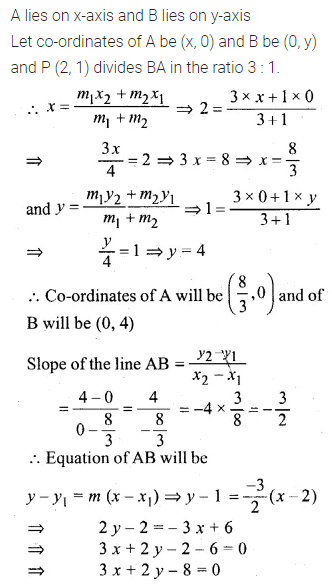Question 15.
A straight line makes on the co-ordinates axes positive intercepts whose sum is 7. If the line passes through the point (-3, 8), find its equation.
Solution:Question 16.
If the coordinates of the vertex A of a square ABCD are (3, -2) and the equestion of diagonal BD is 3x – 7y + 6 = 0, find the equation of the diagonal AC. Also, find the co-ordinates of the centre of the square.
Solution: